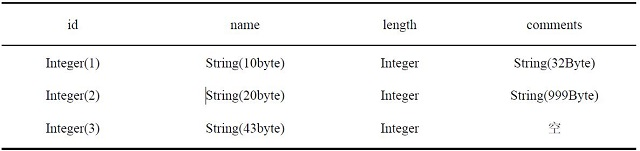Tablestore charges data storage fees on an hourly basis. The total size of data stored in Tablestore tables changes in real time. Tablestore collects statistics on the total size of data stored in Tablestore tables at pre-determined intervals, calculates average total size per hour, and calculates fees by multiplying the average total size per hour by the unit price. The unit price may change. For more information about pricing, see Tablestore Pricing.

The total data size of an instance indicates the size of the data stored in all tables of the instance. The table size is the total size of all rows of data in the table. The following example describes how to calculate the size of data in a table.

Example: A table contains the id primary key column and the name, length, and comments columns.• The size of the row whose value in the id column is 1 is calculated based on the following formula: Size of the row whose value in the id column is 1 = len ('id') + len ('name') + len ('length') + len ('comments'). In other words, size of the row whose value in the id column is 1 is the sum of 8, 10, 8, and 32 bytes, which equals 58 bytes.
• Size of the row whose value in the id column is 2 is calculated based on the following formula: Size of the row whose value in the id column is 2 = len ('id') + len ('name') + len ('length') + len ('comments'). In other words, size of the row whose value in the id column is 2 is the sum of 8, 20, 8, and 999, which equals 1,035 bytes.
• Size of the row whose value in the id column is 3 is calculated based on the following formula: Size of the row whose value in the id column is 3 = len ('id') + len ('name') + len ('length'). In other words, size of the row whose value in the id column is 3 is the sum of 8, 43, and 8, which equals 59 bytes.
• The table size is the sum of 58, 1035, and 59, which equals 1,152 bytes.

If the table size remains unchanged within one hour, fees are calculated based on 1,152 bytes. Tablestore does not impose limits on the amount of data that can be stored in a single table. You can store as much data as necessary. You are charged for the resources you use.